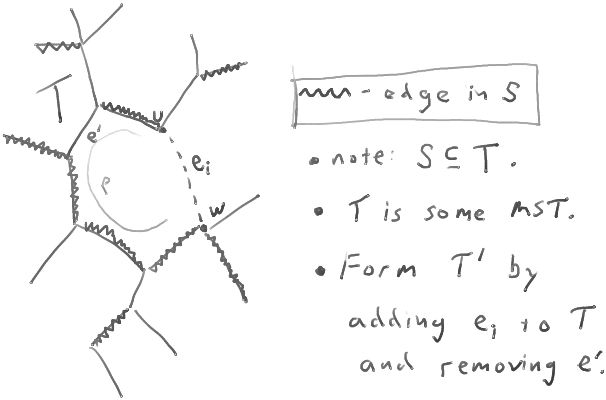# MinimumSpanningTreesByKruskals

ClassS04CS141 | recent changes | Preferences

### Minimum spanning trees

A spanning tree of an undirected graph is a tree containing all vertices in the graph.

If the graph has weights on the edges, the weight of a spanning tree is the total weight of edges on it.

A minimum spanning tree (MST) is a spanning tree of minimum possible weight.

The minimum spanning tree problem is, given a weighted graph, to find a minimum spanning tree.

### Kruskal's algorithm

``` 1.  Sort the edges by weight: e1, e2, ..., em.
2.  S = ∅.
3.  For i=1,2,...,m
4.    If adding ei to S would not create a cycle in S,
5.    then add ei to S.
6.  Return S.
```

To determine whether adding an edge (u,v) would create a cycle, we keep track of the connected components of S using the UnionFind data structure. Here is the code with the augmented with the appropriate use of that data structure.

``` 1.  For each vertex v, makeset(v).
2.  Sort the edges by weight: e1, e2, ..., em.
3.  S = ∅.
4.  For i=1,2,...,m
5.    Let (u,w) = ei.
6.    If find(u) ≠ find(w),
7.    then add ei to S and union(find(u),find(w)).
8.  Return S.
```

#### running time

Sorting the edges takes O(m log m) = O(m log n) time.

There are O(m) unions and finds, these require O(m log n) time.

Thus, the total running time is O(m log n).

#### correctness

(note: the proof in section 7.3.1 of GoodrichAndTomassia is flawed.)

We need to convince ourselves that the set of edges returned by the algorithm forms a minimum spanning tree.

claim: Provided there exists a minimum spanning tree, the algorithm maintains the following invariant: at all times, the set of edges S may be extended to form a minimum spanning tree. (That is, the set S is contained in some MST.)

Note: an "invariant" is just a condition that an algorithm maintains as it runs.

proof of claim: The claim is true initially, when the set S is empty: as long as the graph has an MST, the empty set of edges can be extended by adding the edges in that MST.

Now we need to convince ourselves that the algorithm maintains the invariant when it adds an edge to S. So, suppose the invariant is true (S is contained in some MST T), and then the algorithm adds some edge ei to S. We need to convince ourselves that S ∪ {ei} is contained in some MST T'.

If ei is in T, then we're fine. S∪{ei} can be extended to an MST by adding the edges remaining in T.

Otherwise, let (u,w) = ei. Since T is connected, T contains some path p from u to w:?

Since adding (u,w) to S does not create a cycle, there must be some edge e' on the path p that is not in S.

Since there is no cycle in T, and S is contained in T, if e' had already been considered by the algorithm, the algorithm would have added it to S (since e' could not have created a cycle with the edges already in S). Thus, the edge e' has not yet been considered by the algorithm. Thus, the edge e' has WT(e') ≥ WT(ei.

Form the tree T' by removing e' from T and adding ei. Then T' is a spanning tree, and WT(T') ≤ WT(T). Since T was an MST, it follows that T' is also an MST. Furthermore, T' contains S∪ {ei}. Thus, the set of edges S∪ {ei} can also be extended to form an MST.

This proves the claim.

Now we know that the final set S of edges returned by the algorithm is contained in some minimum spanning tree.

So, to convince ourselves that S is in fact a minimum spanning tree, we need only convince ourselves that S connects the graph.

Suppose for contradiction that there are two vertices u and w that are not reachable from each other using edges in S. Let C be the set of vertices reachable from u. Since the graph is connected, some edge has one endpoint in C and the other endpoint not in C. But when the algorithm considered adding that edge to S, it would have, because that edge did not create a cycle with the edges then in S.

This completes the proof that the algorithm returns an MST.

#### References

ClassS04CS141 | recent changes | Preferences